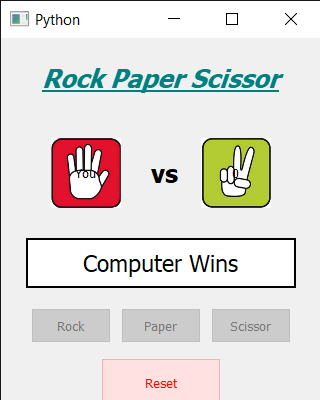Related Articles

# PyQt5 – Rock Paper and Scissor Game

• Last Updated : 03 Jul, 2020

In this article we will see how we can create a rock paper and scissor game using PyQt5. Rock paper scissors is a hand game usually played between two people, in which each player simultaneously forms one of three shapes with an outstretched hand. These shapes are “rock”, “paper”, and “scissors”. Below is the representation of how the game will look like.GUI implementation steps :
1. Create a head label that will show the title of the game, set its font and properties
2. Below the head label create a user label that will show the hand sign selected by user
3. Create a computer label that will show hand sign picked by the computer
4. In between the user and the computer label create a label to show text “vs”
5. Create a result label to show the result set font and other properties to it
6. Create three push buttons for rock, paper and scissor respectively
7. Create a reset button to reset the game

Back end implementation steps :
1. Create user choice and counter variable set its value to -1
2. Add actions to the rock, paper and scissor button
3. Inside the actions set the choice value according to the button pressed and set the counter value to 3 and make all the three button disable
4. Create timer object that calls the method after every one second
5. Inside the timer method check if the counter value is -1 then do nothing else set the counter value to the computer label and decrement the counter
6. And check if the counter value is equal to 0 then get a random value from 1 to 3, according to value set the hand symbol to the computer label
7. Call the who_win method to get the result
8. Inside the who_wins method first check if the match is draw else find the winner and set the winner to the result label
9. Add action to the reset button
10. Inside the reset button action, set counter value to -1, enable all the buttons and remove the image from the computer and user label

Below is the implementation

 `# importing libraries``from` `PyQt5.QtWidgets ``import` `*` `from` `PyQt5 ``import` `QtCore, QtGui``from` `PyQt5.QtGui ``import` `*` `from` `PyQt5.QtCore ``import` `*` `import` `random``import` `sys`` ` ` ` `class` `Window(QMainWindow):`` ` `    ``def` `__init__(``self``):``        ``super``().__init__()`` ` `        ``# setting title``        ``self``.setWindowTitle(``"Python "``)`` ` `        ``# setting geometry``        ``self``.setGeometry(``100``, ``100``, ``320``, ``400``)`` ` `        ``# calling method``        ``self``.UiComponents()`` ` `        ``# showing all the widgets``        ``self``.show()`` ` `    ``# method for components``    ``def` `UiComponents(``self``):`` ` `        ``# counter variable``        ``self``.counter ``=` `-``1`` ` `        ``# choice variable``        ``self``.choice ``=` `0`` ` `        ``# creating head label``        ``head ``=` `QLabel(``"Rock Paper Scissor"``, ``self``)`` ` `        ``# setting geometry to the head``        ``head.setGeometry(``20``, ``10``, ``280``, ``60``)`` ` `        ``# font``        ``font ``=` `QFont(``'Times'``, ``15``)``        ``font.setBold(``True``)``        ``font.setItalic(``True``)``        ``font.setUnderline(``True``)`` ` `        ``# setting font to the head``        ``head.setFont(font)`` ` `        ``# setting alignment of the head``        ``head.setAlignment(Qt.AlignCenter)`` ` `        ``# setting color effect to the head``        ``color ``=` `QGraphicsColorizeEffect(``self``)``        ``color.setColor(Qt.darkCyan)``        ``head.setGraphicsEffect(color)`` ` `        ``# creating a vs label``        ``self``.vs ``=` `QLabel(``"vs"``, ``self``)`` ` `        ``# setting geometry``        ``self``.vs.setGeometry(``150``, ``110``, ``30``, ``50``)`` ` `        ``# setting font``        ``font.setUnderline(``False``)``        ``font.setItalic(``False``)``        ``self``.vs.setFont(font)`` ` `        ``# creating your choice label``        ``self``.user ``=` `QLabel(``"You"``, ``self``)`` ` `        ``# setting geometry``        ``self``.user.setGeometry(``50``, ``100``, ``70``, ``70``)``        ``self``.user.setStyleSheet(``"border : 2px solid black; background : white;"``)`` ` `        ``# setting alignment``        ``self``.user.setAlignment(Qt.AlignCenter)`` ` `        ``# creating computer choice  label``        ``self``.computer ``=` `QLabel(``"Computer"``, ``self``)`` ` `        ``# setting geometry``        ``self``.computer.setGeometry(``200``, ``100``, ``70``, ``70``)``        ``self``.computer.setStyleSheet(``"border : 2px solid black; background : white;"``)`` ` `        ``# setting alignment``        ``self``.computer.setAlignment(Qt.AlignCenter)`` ` `        ``# result label``        ``self``.result ``=` `QLabel(``self``)`` ` `        ``# setting geometry to the result``        ``self``.result.setGeometry(``25``, ``200``, ``270``, ``50``)`` ` `        ``# setting font``        ``self``.result.setFont(QFont(``'Times'``, ``14``))`` ` `        ``# setting alignment``        ``self``.result.setAlignment(Qt.AlignCenter)`` ` `        ``# setting border and color``        ``self``.result.setStyleSheet(``"border : 2px solid black; background : white;"``)`` ` ` ` `        ``# creating three push button``        ``# for rock paper and scissor``        ``self``.rock ``=` `QPushButton(``"Rock"``, ``self``)``        ``self``.rock.setGeometry(``30``, ``270``, ``80``, ``35``)`` ` `        ``self``.paper ``=` `QPushButton(``"Paper"``, ``self``)``        ``self``.paper.setGeometry(``120``, ``270``, ``80``, ``35``)`` ` `        ``self``.scissor ``=` `QPushButton(``"Scissor"``, ``self``)``        ``self``.scissor.setGeometry(``210``, ``270``, ``80``, ``35``)`` ` `        ``# adding actions to the buttons``        ``self``.rock.clicked.connect(``self``.rock_action)``        ``self``.paper.clicked.connect(``self``.paper_action)``        ``self``.scissor.clicked.connect(``self``.scissor_action)`` ` `        ``# creating push button to reset all the game``        ``game_reset ``=` `QPushButton(``"Reset"``, ``self``)`` ` `        ``# setting geometry``        ``game_reset.setGeometry(``100``, ``320``, ``120``, ``50``)`` ` `        ``# setting color effect``        ``color ``=` `QGraphicsColorizeEffect(``self``)``        ``color.setColor(Qt.red)``        ``game_reset.setGraphicsEffect(color)`` ` `        ``# adding action tot he reset button``        ``game_reset.clicked.connect(``self``.reset_action)`` ` `        ``# creating a timer object``        ``timer ``=` `QTimer(``self``)`` ` `        ``# adding action to the timer``        ``timer.timeout.connect(``self``.showTime)`` ` `        ``# starting the timer``        ``timer.start(``1000``)`` ` ` ` ` ` `    ``def` `showTime(``self``):`` ` `        ``# if counter value is - 1``        ``if` `self``.counter ``=``=` `-``1``:``            ``pass`` ` `        ``# if counter is not - 1``        ``else``:``             ` `            ``# setting counter value to the label``            ``self``.computer.setText(``str``(``self``.counter))`` ` `            ``if` `self``.counter ``=``=` `0``:``                ``self``.comp_choice ``=` `random.randint(``1``, ``3``)`` ` `                ``# if computer choice is 1``                ``if` `self``.comp_choice ``=``=` `1``:`` ` `                    ``# setting rock image to the computer label``                    ``self``.computer.setStyleSheet(``"border-image : url(rock.png);"``)`` ` `                ``elif` `self``.comp_choice ``=``=` `2``:``                    ``# setting paper image to the computer label``                    ``self``.computer.setStyleSheet(``"border-image : url(Paper.png);"``)`` ` `                ``else``:``                    ``# setting scissor image to the computer label``                    ``self``.computer.setStyleSheet(``"border-image : url(scissor.png);"``)`` ` `                ``# checking who won the match``                ``self``.who_won()`` ` `            ``# decrementing the counter value``            ``self``.counter ``-``=` `1`` ` ` ` `    ``def` `rock_action(``self``):`` ` `        ``# making choice as 1``        ``self``.choice ``=` `1`` ` `        ``# setting rock image to the user label``        ``self``.user.setStyleSheet(``"border-image : url(rock.png);"``)`` ` `        ``# making counter value to 3``        ``self``.counter ``=` `3`` ` `        ``# disabling the push button``        ``self``.rock.setDisabled(``True``)``        ``self``.paper.setDisabled(``True``)``        ``self``.scissor.setDisabled(``True``)`` ` `    ``def` `paper_action(``self``):`` ` `        ``# making choice as 2``        ``self``.choice ``=` `2`` ` `        ``# setting rock image to the user label``        ``self``.user.setStyleSheet(``"border-image : url(Paper.png);"``)`` ` `        ``# making counter value to 3``        ``self``.counter ``=` `3`` ` `        ``# disabling the push button``        ``self``.rock.setDisabled(``True``)``        ``self``.paper.setDisabled(``True``)``        ``self``.scissor.setDisabled(``True``)`` ` `    ``def` `scissor_action(``self``):`` ` `        ``# making choice as 3``        ``self``.choice ``=` `3`` ` `        ``# setting rock image to the user label``        ``self``.user.setStyleSheet(``"border-image : url(scissor.png);"``)`` ` `        ``# making counter value to 3``        ``self``.counter ``=` `3`` ` `        ``# disabling the push button``        ``self``.rock.setDisabled(``True``)``        ``self``.paper.setDisabled(``True``)``        ``self``.scissor.setDisabled(``True``)`` ` ` ` `    ``def` `reset_action(``self``):`` ` `        ``# making result label empty``        ``self``.result.setText("")`` ` `        ``# resting the counter value``        ``self``.counter ``=` `-``1`` ` `        ``# enabling the push buttons``        ``self``.rock.setEnabled(``True``)``        ``self``.paper.setEnabled(``True``)``        ``self``.scissor.setEnabled(``True``)`` ` `        ``# removing images fro the user and computer label``        ``self``.user.setStyleSheet(``"border-image : null;"``)``        ``self``.computer.setStyleSheet(``"border-image : null;"``)`` ` ` ` `    ``def` `who_won(``self``):`` ` `        ``# if match is draw``        ``if` `self``.choice ``=``=` `self``.comp_choice:`` ` `            ``# setting text to the result label``            ``self``.result.setText(``"Draw Match"``)`` ` `        ``else``:``            ``# condition for winning``            ``# user choose rock``            ``if` `self``.choice ``=``=` `1``:``                ``# computer choose paper``                ``if` `self``.comp_choice ``=``=` `2``:``                    ``# setting text to the result``                    ``self``.result.setText(``"Computer Wins"``)``                ``else``:``                    ``self``.result.setText(``"User Wins"``)`` ` `            ``# user chooses paper``            ``elif` `self``.choice ``=``=` `2``:``                ``# computer choose scissor``                ``if` `self``.comp_choice ``=``=` `3``:``                    ``# setting text to the result``                    ``self``.result.setText(``"Computer Wins"``)``                ``else``:``                    ``self``.result.setText(``"User Wins"``)`` ` `            ``# if user chooses scissor``            ``elif` `self``.choice ``=``=` `3``:``                ``# computer choose rock``                ``if` `self``.comp_choice ``=``=` `1``:``                    ``# setting text to the result``                    ``self``.result.setText(``"Computer Wins"``)``                ``else``:``                    ``self``.result.setText(``"User Wins"``)`` ` ` ` ` ` ` ` ` ` `# create pyqt5 app``App ``=` `QApplication(sys.argv)`` ` `# create the instance of our Window``window ``=` `Window()`` ` `# start the app``sys.exit(App.``exec``())`

Output :

Attention geek! Strengthen your foundations with the Python Programming Foundation Course and learn the basics.

To begin with, your interview preparations Enhance your Data Structures concepts with the Python DS Course. And to begin with your Machine Learning Journey, join the Machine Learning – Basic Level Course

My Personal Notes arrow_drop_up